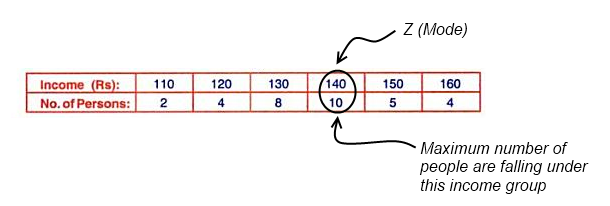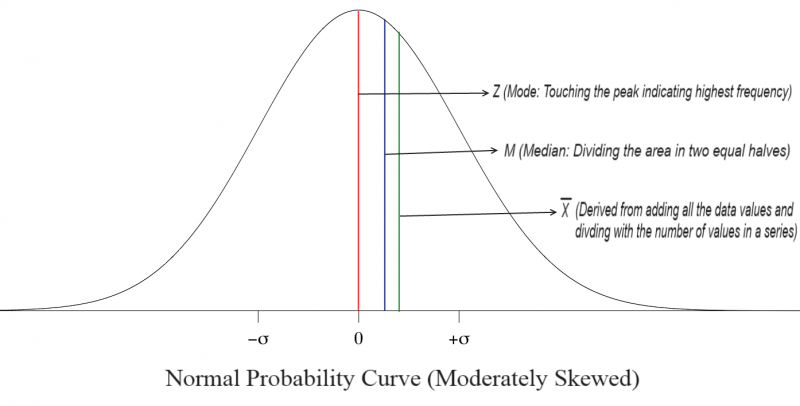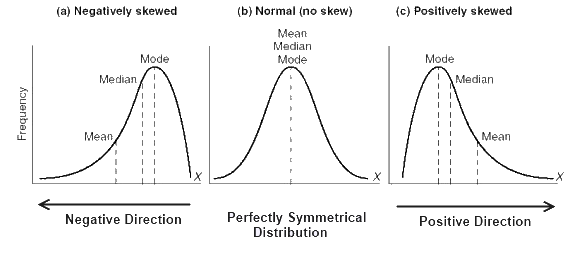The word mode is derived from a French word “La Mode” which means a thing in fashion. Therefore, mode is a value that occurs most frequently in the series.

In statistics, it is represented by the letter Z.

The easiest way to find out mode is through inspection.

E.g.Merits of Mode:

1. Only a glance is enough to figure out the modal value.
2. It is also the best representative of the series as it shows that value, which occurs most frequently in the series.
3. Used for categorical data. Used where mean and median can’t be used.

Demerits of Mode:

1. Can’t be used for further mathematical treatments.
2. There is no continuity in the data and it ignores the rest of the data completely.

In a normal distribution curve, ideally, the mean, median and mode should fall in the same place and in the centre (Mean= Median= Mode). However, it is almost impossible for the distribution to be perfectly symmetrical. If it is moderately asymmetrical distribution the empirical relationship between the three holds good. In such distributions the distance between the mean and median is about one-third of the distance between the mean and mode.

Mode= 3 Median – 2 MeanIf it is positively skewed mean > median > mode.

If it is negatively skewed mode > median > mean.Share.